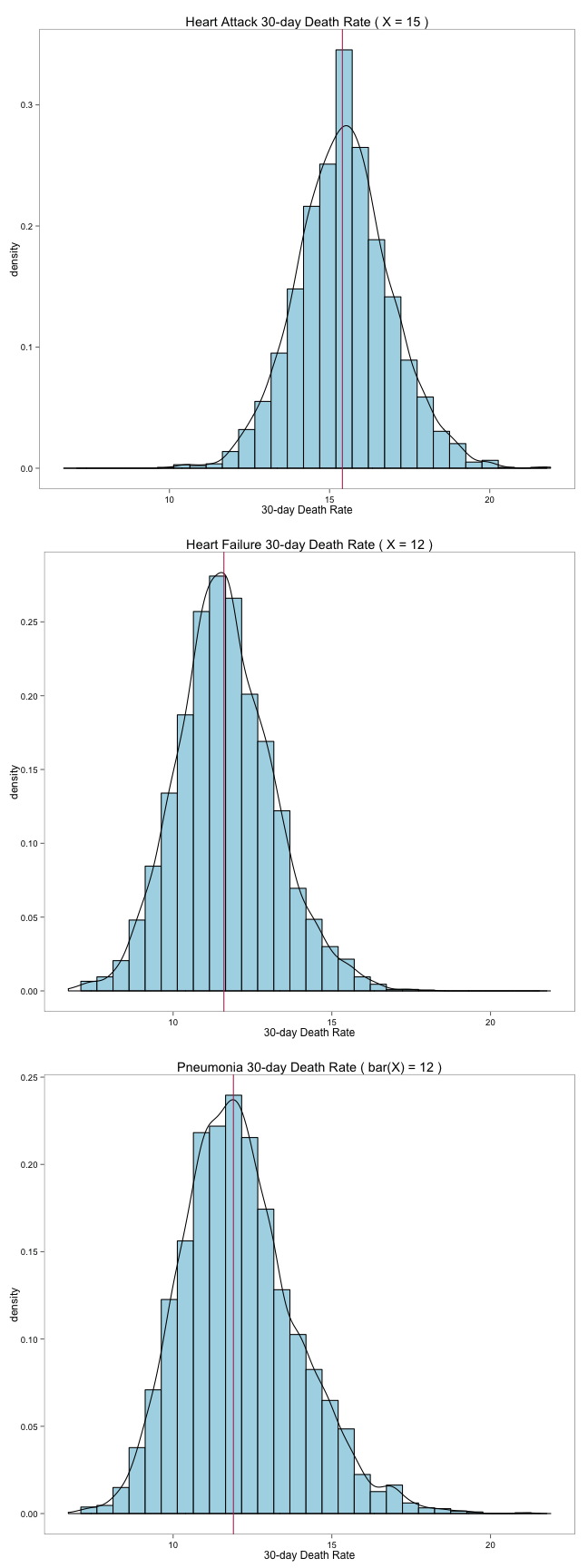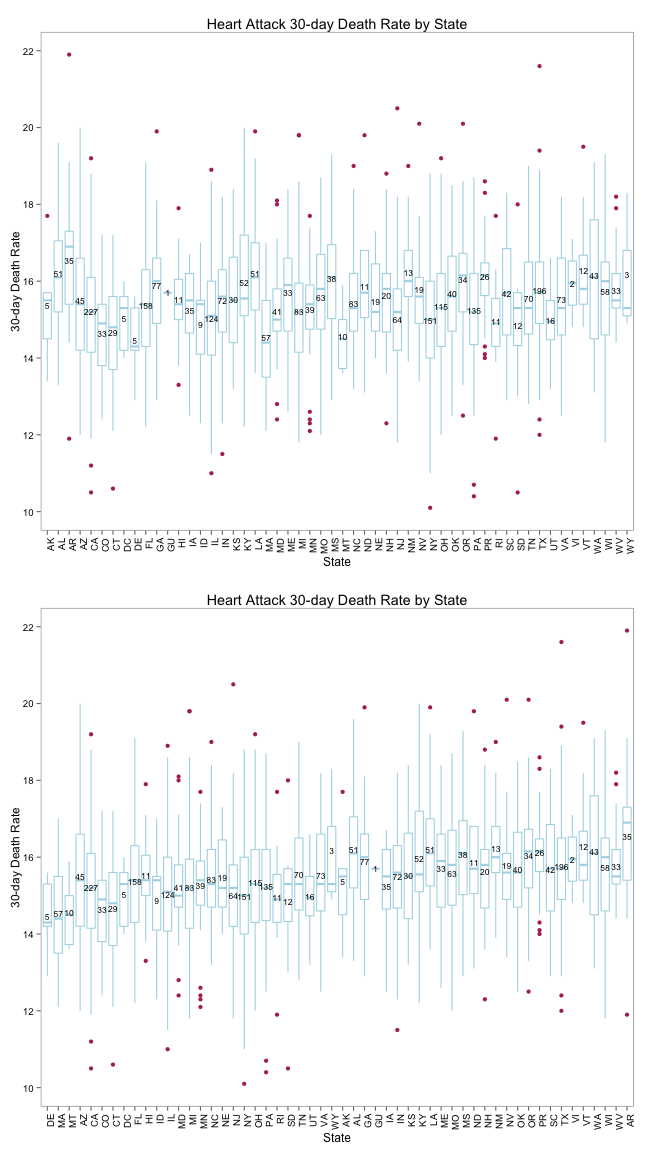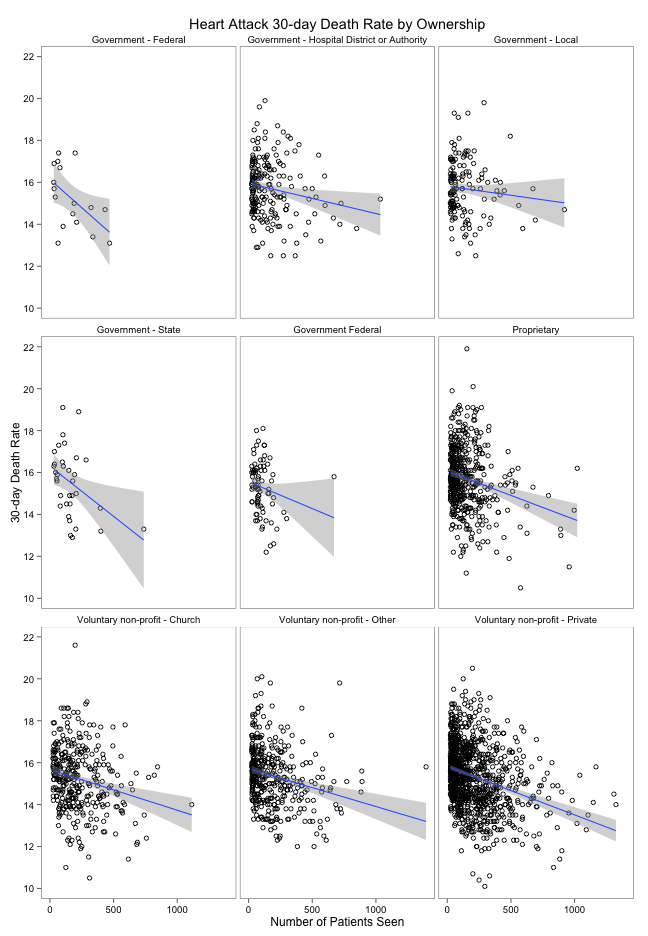# Coursera's “Computing For Data Analysis” Programming Assignment 3 Graphs Using ggplot2

The base graphics package in R is powerful and flexible, but has some quriks, inconsistencies and other issues. The ggplot2 package aims to “take the good parts of base and lattice graphics and none of the bad parts.”

Two funamentals of ggplot are that it works off of data frames – making it more straightforward to use with a wide variety of workflows in R – and is based on the grammar of graphics.

It can handle much of the dirty work for you and can make very visually appealing charts with little effort.

I thought it would be an interesting exercise to

We'll be using the following libraries (there's a short description next to each of them):

``````library(ggplot2)  # need this to use'ggplot'
library(ggthemes)  # many additional beautiful themes
library(gridExtra)  # replaces 'par()'
``````

We still need to do the data work..

``````df = read.csv("~/Desktop/p3/outcome-of-care-measures.csv", colClasses = "character")

# I like to work with column names vs indicies

colnames(df) = "HeartAttack"
colnames(df) = "HeartFailure"
colnames(df) = "Pneumonia"

# convert everything we need to numbers

df\$HeartAttack = as.numeric(df\$HeartAttack)
df\$HeartFailure = as.numeric(df\$HeartFailure)
df\$Pneumonia = as.numeric(df\$Pneumonia)

# compute the ranges of each column

rHA = range(df\$HeartAttack, na.rm = TRUE)
rHF = range(df\$HeartFailure, na.rm = TRUE)
rP = range(df\$Pneumonia, na.rm = TRUE)

# get min/max for xlim

rng = c(min(c(rHA, rHF, rP)), max(c(rHA, rHF, rP)))

# get mean values

meanHeartAttack = round(mean(df\$HeartAttack, na.rm = TRUE))
meanHeartFailure = round(mean(df\$HeartFailure, na.rm = TRUE))
meanPneumonia = round(mean(df\$Pneumonia, na.rm = TRUE))
``````

Here's where the ggplot work comes in. We build three graphs of the death rate for each condition and put them into a grid.

I like to put each ggplot element on a separate line as I'm building a plot, and that also makes it easier to explain what's going on:

• You can see that we start by calling `ggplot()` and tell it which data frame (`df`) we're working with.
• We then tell it we're using the heart attack death rate data and that we want to plot a histogram (using density vs frequency).
• We then layer the smooth density estimate line over the histogram.
• And, then plot the median value.
• We set the labels.
• And, modify the scale (as instructed).
• I added the `theme_few()` to make it prettier than the ggplot defaults (try it without that line)

Lather/rinse/repeat for each death reate.

``````haPlot = ggplot(df, aes(x = HeartAttack)) + geom_histogram(aes(y = ..density..),
fill = "lightblue", color = "black") + geom_density() + geom_vline(xintercept = median(df\$HeartAttack,
na.rm = TRUE), color = "maroon") + labs(title = paste("Heart Attack 30-day Death Rate ( X =",
meanHeartAttack, ")"), x = "30-day Death Rate") + scale_x_continuous(limits = rng) +
theme_few()

hfPlot = ggplot(df, aes(x = HeartFailure)) + geom_histogram(aes(y = ..density..),
fill = "lightblue", color = "black") + geom_density() + geom_vline(xintercept = median(df\$HeartFailure,
na.rm = TRUE), color = "maroon") + labs(title = paste("Heart Failure 30-day Death Rate ( X =",
meanHeartFailure, ")"), x = "30-day Death Rate") + scale_x_continuous(limits = rng) +
theme_few()

pnPlot = ggplot(df, aes(x = Pneumonia)) + geom_histogram(aes(y = ..density..),
fill = "lightblue", color = "black") + geom_density() + geom_vline(xintercept = median(df\$Pneumonia,
na.rm = TRUE), color = "maroon") + labs(title = paste("Pneumonia 30-day Death Rate ( bar(X) =",
meanPneumonia, ")"), x = "30-day Death Rate") + scale_x_continuous(limits = rng) +
theme_few()
``````

We then use `grid.arrange()` to mimic the `par()` function to show our work:

``````grid.arrange(haPlot, hfPlot, pnPlot, nrow = 3)
``````Next, we'll show the boxplots from the assignment, and I'll show the sorted & non-sorted ones together. I'm ignoring the math symbol requirement as it seemed to be a rather silly focus for a data analysis course.

I've annotated the R code pretty well, so no need to explain more here.

``````# get median values and merge back into the data frame

dfHAMed = with(df, aggregate(HeartAttack, by = list(State), FUN = function(v) {
round(median(v, na.rm = TRUE))
}))
colnames(dfHAMed) = c("State", "Median.Heart.Attack.Death.Rate")
df = merge(df, dfHAMed, by = "State")

# since we're going to show the sorted & non-sorted plots together make
# another data frame with the sorted values vs sort in place

df.sort1 = transform(df, State = reorder(State, Median.Heart.Attack.Death.Rate))

# this is a function that we'll use in stat_summary() which will provide
# the population size for the state at the median line (as I'm not a fan
# of altering the x axis labels with this info)

lengthformean <- function(x) {
return(c(y = mean(x), label = length(x)))
}
``````

Now, we enerate the sorted & non-sorted box plots for use with the grid plot. Again, we store these in varibles (_ggplot_s are just objects) for actual plotting later on.

• We start again telling `ggplot()` what data frame we're using and which bits to use for `x` & `y` values.
• We tell it to do a box plot
• We set the labels
• We use `stat_summary()` to show the population size right at the mean line of the box plot boxes
• We make it pretty
• We rotate the text (just to show you how)
``````bystate = ggplot(df, aes(factor(State), HeartAttack)) + geom_boxplot(outlier.colour = "maroon",
fill = "white", color = "lightblue") + labs(x = "State", y = "30-day Death Rate",
title = "Heart Attack 30-day Death Rate by State") + stat_summary(fun.data = lengthformean,
geom = "text", color = "black", size = 3, position = "stack") + theme_few() +
theme(axis.text.x = element_text(angle = 90, hjust = 1))

bymedian = ggplot(df.sort1, aes(factor(State), HeartAttack)) + geom_boxplot(outlier.colour = "maroon",
fill = "white", color = "lightblue") + labs(x = "State", y = "30-day Death Rate",
title = "Heart Attack 30-day Death Rate by State") + stat_summary(fun.data = lengthformean,
geom = "text", color = "black", size = 3, position = "stack") + theme_few() +
theme(axis.text.x = element_text(angle = 90, hjust = 1))
``````

Finally, we plot the figures

``````grid.arrange(bystate, bymedian, nrow = 2)
``````Now we're ready to compare the 30-day death rates and numbers of patients with latticed scatterplots.

``````# read in the additional data
hospital = read.csv("~/Desktop/p3/hospital-data.csv", colClasses = "character")

# merge the old & new data frames together
outcome.hospital = merge(df, hospital, by = "Provider.Number")

# I like decent column names to work with
colnames(outcome.hospital) = "death"
colnames(outcome.hospital) = "npatient"

# Make sure the necessary column are numeric (read in as strings,
# remember)
outcome.hospital\$death = as.numeric(outcome.hospital\$death)
outcome.hospital\$npatient = as.numeric(outcome.hospital\$npatient)
``````

The ggplot2 library is nothing short of magic when it comes to how succinctly we can make complex graphs. For the latticed scatterplot, we:

• Tell `ggplot()` what our data frame is and what our columns are for `x` & `y`
• Say that we want to use hollow circles
• Add a linear regression line
• Take what would normally be a long row of panels (since we're plotting many scatterplots by a particular variable) and wrap it into a grid
• Set labels
• Make it pretty
``````sc = ggplot(outcome.hospital, aes(x = npatient, y = death)) + geom_point(shape = 1) +
geom_smooth(method = lm) + facet_wrap(~Hospital.Ownership) + labs(x = "Number of Patients Seen",
y = "30-day Death Rate", title = "Heart Attack 30-day Death Rate by Ownership") +
theme_few()
``````
``````sc
``````